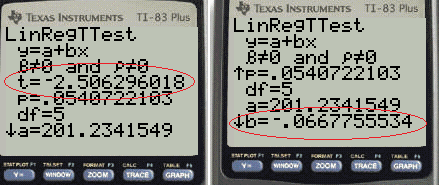# Standard Error of Regression Slope

Share on

## Standard Error of Regression Slope: Overview

Standard errors for regression are measures of how spread out your y variables are around the mean, μ.The standard error of the regression slope, s (also called the standard error of estimate) represents the average distance that your observed values deviate from the regression line. The smaller the “s” value, the closer your values are to the regression line.

Watch the video for an overview of standard errors in linear regression, including SE for coefficients and the slope. The video also shows how to calculate the standard error for the slope/coefficient in Excel:

Standard error of regression slope is a term you’re likely to come across in AP Statistics. In fact, you’ll find the formula on the AP statistics formulas list given to you on the day of the exam.

### Standard Error of Regression Slope Formula / TI-83 Instructions

SE of regression slope = sb1 = sqrt [ Σ(yi – ŷi)2 / (n – 2) ] / sqrt [ Σ(xix)2 ].

The equation looks a little ugly, but the secret is you won’t need to work the formula by hand on the test. Even if you think you know how to use the formula, it’s so time-consuming to work that you’ll waste about 20-30 minutes on one question if you try to do the calculations by hand! The TI-83 calculator is allowed in the test and it can help you find the standard error of regression slope.

Note: The TI83 doesn’t find the SE of the regression slope directly; the “s” reported on the output is the SE of the residuals, not the SE of the regression slope. However, you can use the output to find it with a simple division.

Step 1: Enter your data into lists L1 and L2. If you don’t know how to enter data into a list, see:TI-83 Scatter Plot.)

Step 2: Press , scroll right to TESTS and then select E:LinRegTTest

Step 3: Type in the name of your lists into the Xlist and Ylist. For example, type L1 and L2 if you entered your data into list L1 and list L2 in Step 1.

Step 4: Select the sign from your alternate hypothesis. For example, select (≠ 0) and then press .

Step 5: Highlight Calculate and then press .

Step 6: Find the “t” value and the “b” value. You may need to scroll down with the arrow keys to see the result. For example, let’s sat your t value was -2.51 and your b value was -.067.Step 7: Divide b by t. For this example, -0.67 / -2.51 = 0.027.

The standard error of regression slope for this example is 0.027.

That’s it!

## References

Beyer, W. H. CRC Standard Mathematical Tables, 31st ed. Boca Raton, FL: CRC Press, pp. 536 and 571, 2002.
Everitt, B. S.; Skrondal, A. (2010), The Cambridge Dictionary of Statistics, Cambridge University Press.
Kotz, S.; et al., eds. (2006), Encyclopedia of Statistical Sciences, Wiley.
Wheelan, C. (2014). Naked Statistics. W. W. Norton & Company

CITE THIS AS:
Stephanie Glen. "Standard Error of Regression Slope" From StatisticsHowTo.com: Elementary Statistics for the rest of us! https://www.statisticshowto.com/probability-and-statistics/regression-analysis/find-standard-error-regression-slope/
---------------------------------------------------------------------------Need help with a homework or test question? With Chegg Study, you can get step-by-step solutions to your questions from an expert in the field. Your first 30 minutes with a Chegg tutor is free!